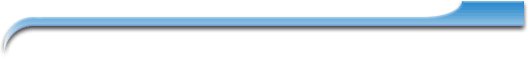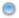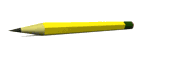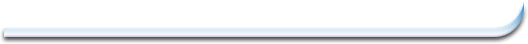Resources Links Gallery Marks: MCR 3UE P#1 MTH 1MP P#2 MTH 1M2 P#3 E-MailResourcesCourse Outlines and Homework:

AP Calc     Syllabus - here     Work - here

MCV 4UP       Outline - here      Work - here

MHF 4UP       Outline - here      Work - here

MCR 3UP      Outline - here      Work - here       Brebeuf Outline - here

MPM 2DP      Outline - here      Work - here       Brebeuf Outline - here

MPM 1M2-P  Outline - here      Work - here       Brebeuf Outline - here

Mathematics Resources:

Grade 9:  Perimeter, Area, Surface Area, Volume  -  here

Grade 11:  Financial Formulas -  here

Parent Functions -  here

Transforming Parent Function Graphs -  here

Trigonometric Formulas and Unit Circle -  here

Trigonometric Blank Sketch Axis -  here

Trigonometric Known Values  -  here

Grade 12:  Logarithmic Formulas -  here

Calculus Curve Sketching Routine -  here

Calculus Limit Rules -  here

Calculus Vector Formulas -  here

Vectors Points-Lines-Planes -  here

Ask If you need More Resources !!